# A Physics Problem Recently Sent to Me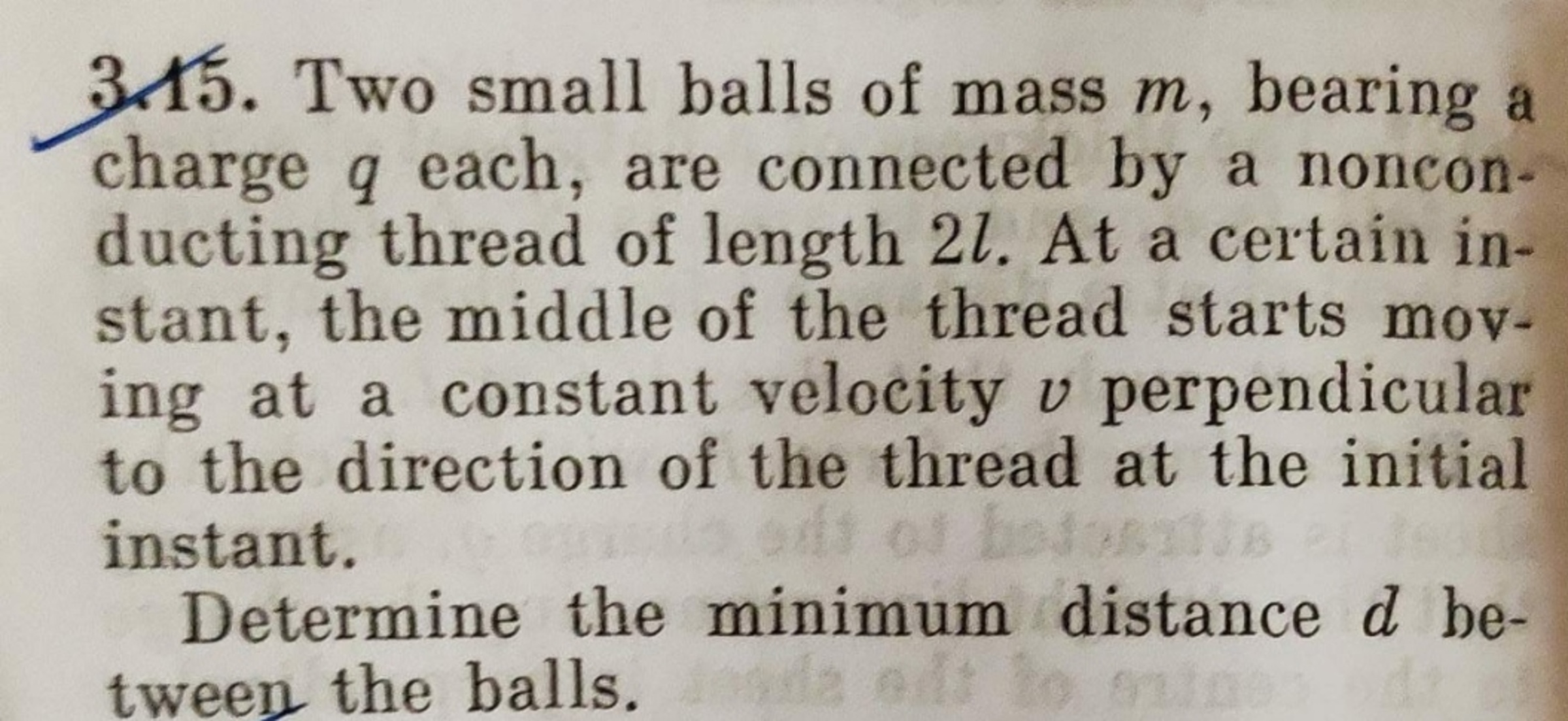I have come to find out that my solution is not the simplest possible one, but here it is anyway.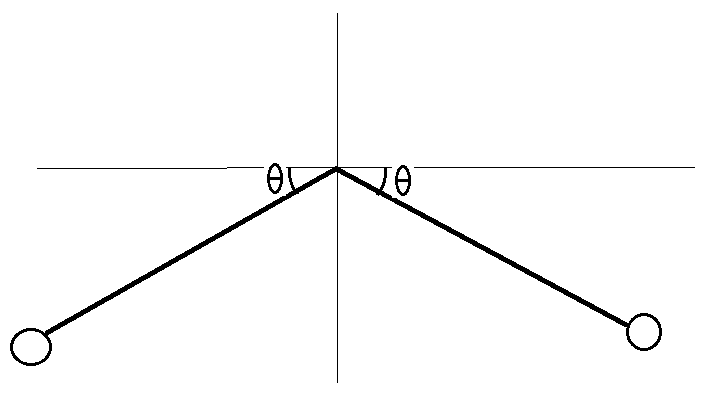Assume that the middle of the string moves with constant speed. The position and velocity of the right ball is:

$x = L \cos \theta \\ y = v t - L \sin \theta \\ \dot{x} = -L \sin \theta \dot{\theta} \\ \dot{y} = v - L cos \theta \dot{\theta}$

The kinetic energy $T$, potential energy $V$, and Lagrangian are:

$T = m L^2 \dot{\theta}^2 + m v^2 - 2 m v L \cos \theta \dot{\theta} \\ V = \frac{k_e q^2}{2 L \cos \theta} = \frac{k_e q^2}{2 L } \sec \theta \\ \mathcal{L} = T - V = m L^2 \dot{\theta}^2 + m v^2 - 2 m v L \cos \theta \dot{\theta} - \frac{k_e q^2}{2 L } \sec \theta$

Equation of motion:

$\frac{d}{dt} \frac{\partial{\mathcal{L}}}{\partial{\dot{\theta}}} = \frac{\partial{\mathcal{L}}}{\partial{\theta}}$

Evaluating these equations results in:

$\ddot{\theta} = - \frac{k_e q^2}{4 m L^3} \sec \theta \tan \theta$

Suppose the physical parameters are such that the oscillations are small. In this case:

$\ddot{\theta} \approx - \frac{k_e q^2}{4 m L^3} \theta \\ \gamma = \sqrt{\frac{k_e q^2}{4 m L^3} }$

This corresponds to simple harmonic motion with angular frequency $\gamma$. Writing out the general form for the solution:

$\theta = A \cos(\gamma t) + B \sin(\gamma t) \\ \dot{\theta} = -A \gamma \sin(\gamma t) + B \gamma \cos(\gamma t)$

Assume that the balls start at rest. Applying initial conditions $\theta(0) = 0$ and $\dot{\theta}(0) = \frac{v}{L}$ results in:

$\theta = \frac{v}{\gamma L} \sin(\gamma t)$

The maximum value of $\theta$ is:

$\theta_{max } = \frac{v}{\gamma L} = \frac{v}{L} \sqrt{\frac{4 m L^3}{k_e q^2}}$

The minimum distance between the charges is:

$D_{min} = 2 L \cos \theta_{max} = 2L \cos \Big(\sqrt{\frac{16 \pi \epsilon_0 m L v^2}{q^2}} \Big)$

We can work this into an alternate form which matches the form expected in an answer key. Since the argument of the cosine function is small (by assumption), we can represent the cosine as a two-term Taylor expansion:

$\cos{\alpha} \approx 1 - \frac{\alpha^2}{2} \\ D_{min} \approx 2 L \Big( 1 - \frac{8 \pi \epsilon_0 m L v^2}{q^2}\Big) = 2 L \Big( \frac{q^2 - 8 \pi \epsilon_0 m L v^2}{q^2}\Big)$

A bit more manipulation:

$D_{min} = 2 L \Big( \frac{q^2 - 8 \pi \epsilon_0 m L v^2}{q^2}\Big) = 2 L \Big( \frac{q^2 - \beta}{q^2}\Big) = 2 L \Big( \frac{q^2 - \beta}{q^2}\Big) \Big(\frac{q^2 + \beta}{q^2 + \beta}\Big) = 2 L \Big( \frac{q^4 - \beta^2}{q^4 + \beta q^2}\Big)$

Since $\beta$ is presumed to be small:

$D_{min} = 2 L \Big( \frac{q^4 - \beta^2}{q^4 + \beta q^2}\Big) \approx 2 L \Big( \frac{q^4}{q^4 + \beta q^2}\Big) = 2 L \Big( \frac{q^2}{q^2 + \beta}\Big) = 2 L \Big( \frac{q^2}{q^2 + 8 \pi \epsilon_0 m L v^2}\Big)$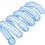Note by Steven Chase
1 year, 1 month ago

This discussion board is a place to discuss our Daily Challenges and the math and science related to those challenges. Explanations are more than just a solution — they should explain the steps and thinking strategies that you used to obtain the solution. Comments should further the discussion of math and science.

When posting on Brilliant:

• Use the emojis to react to an explanation, whether you're congratulating a job well done , or just really confused .
• Ask specific questions about the challenge or the steps in somebody's explanation. Well-posed questions can add a lot to the discussion, but posting "I don't understand!" doesn't help anyone.
• Try to contribute something new to the discussion, whether it is an extension, generalization or other idea related to the challenge.

MarkdownAppears as
*italics* or _italics_ italics
**bold** or __bold__ bold
- bulleted- list
• bulleted
• list
1. numbered2. list
1. numbered
2. list
Note: you must add a full line of space before and after lists for them to show up correctly
paragraph 1paragraph 2

paragraph 1

paragraph 2

[example link](https://brilliant.org)example link
> This is a quote
This is a quote
    # I indented these lines
# 4 spaces, and now they show
# up as a code block.

print "hello world"
# I indented these lines
# 4 spaces, and now they show
# up as a code block.

print "hello world"
MathAppears as
Remember to wrap math in $$ ... $$ or $ ... $ to ensure proper formatting.
2 \times 3 $2 \times 3$
2^{34} $2^{34}$
a_{i-1} $a_{i-1}$
\frac{2}{3} $\frac{2}{3}$
\sqrt{2} $\sqrt{2}$
\sum_{i=1}^3 $\sum_{i=1}^3$
\sin \theta $\sin \theta$
\boxed{123} $\boxed{123}$

Sort by:

@Steven Chase the solution of book which I shared with you was correct or incorrect???
Because according to @Karan Chatrath he said , the solution is incorrect.

- 1 year, 1 month ago

I'm pretty satisfied with the solution. If he would like to comment, I would be happy to hear it.

- 1 year, 1 month ago

Well, I did not say that the solution is incorrect. Please do not misquote me. I said that the solution provided in the book made no sense to me. It was just a few lines with minimal explanation.

Coming to the solution provided by @Steven Chase, I must say that it is pretty elegant. I solved the problem using a Newtonian formulation and by using Cartesian coordinates instead of the $\theta$ coordinate. The resulting equations of motion I obtained were pretty big and I saw no other way to proceed apart from a numerical route.

Thank you for sharing this note.

- 1 year, 1 month ago

@Karan Chatrath Ok sir. Sorry.
Now I have retracted what I said.
I want to ask you a single correct question.

Choose the correct option:

The book solution is
a)Correct
b)Incorrect

It is compulsory to answer the question.

- 1 year, 1 month ago

If I had to choose only among these two options, I choose the second one. This is because the initial speed of each ball is not $v$. The balls are initially separated by $2L$ and are at rest. That is what my solution predicts. Moreover, if you notice the expressions for velocity components provided in this note, they also indicate that the initial speed of each ball is zero unlike the solution provided in the book. Beyond this point, nothing else made sense to me while reading the solution provided in your book.

- 1 year, 1 month ago

One thing to keep in mind is that in the book solution, they take a frame of reference coinciding with the center of the string. In that case, the initial velocity of each ball is $v$.

- 1 year, 1 month ago

Okay, I missed that key phrase, now that you draw attention to it. And this also means that I stand corrected about my stance about the solution being incorrect. But I cannot help but think that even if we were to take an inertial frame of reference at rest, such as I have, the energy conservation principle should yield the answer. I wonder why isn't that so.

- 1 year, 1 month ago

Right, it is not clear whether the mid point is supposed to have constant speed or not. In constant-energy systems with no external forcing functions, all parts generally oscillate or change speed somehow. In my conception of the problem, there is external forcing. See my other comment

- 1 year, 1 month ago

What book is this? I wonder.

- 1 year, 1 month ago

Not sure. All I have is that image

- 1 year, 1 month ago

@Neeraj Anand Badgujar To elaborate further:

Taking $\epsilon_o=m=q=1$, $L=2$, $v=0.1$ and solving the equations of motion (derived in cartesian coordinates) numerically, I get for each ball: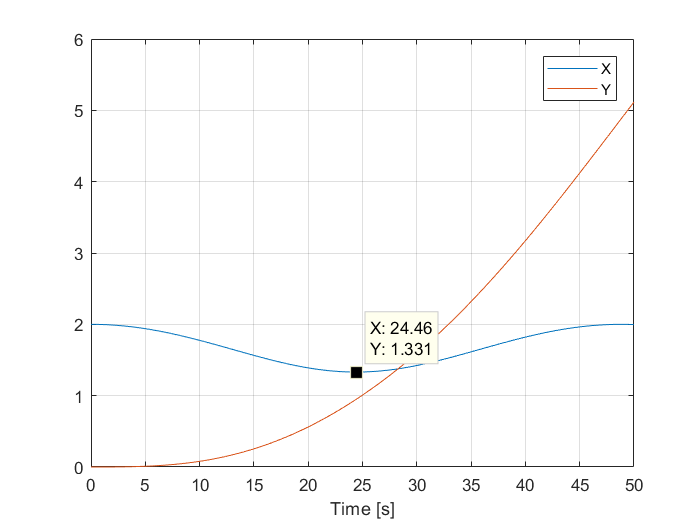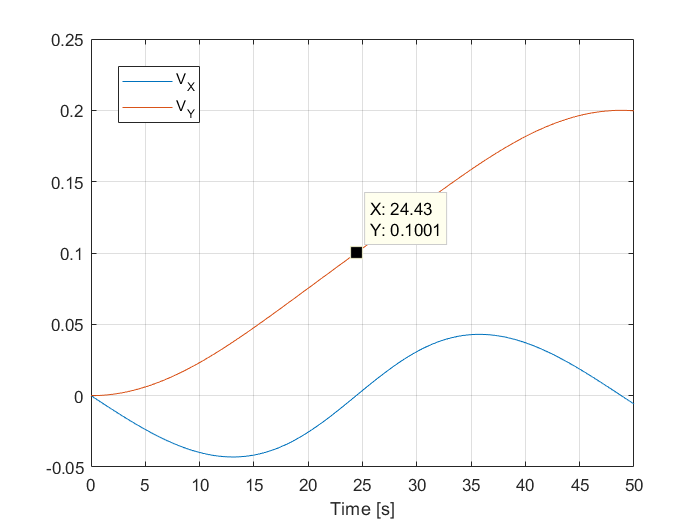Notice the initial speed of each ball is zero. Moreover, at the instant of minimum separation, the y component of the velocity of each ball is 0.1 and the x-component is zero. So using this information, if we were to apply the energy conservation principle, then:

$PE_{initial} + KE_{initial} =PE_{final} + KE_{final}$

$\frac{KQ^2}{2L} = \frac{KQ^2}{d} + mv^2$

This is different from the solution provided in the book. It seems weird to me why the energy conservation principle does not work for the masses here, but I think that the equation of energy conservation must also account for the energy provided to the system due to the prescribed motion.

@Steven Chase what do you think? For reference, I am attaching the snapshot shared with me earlier.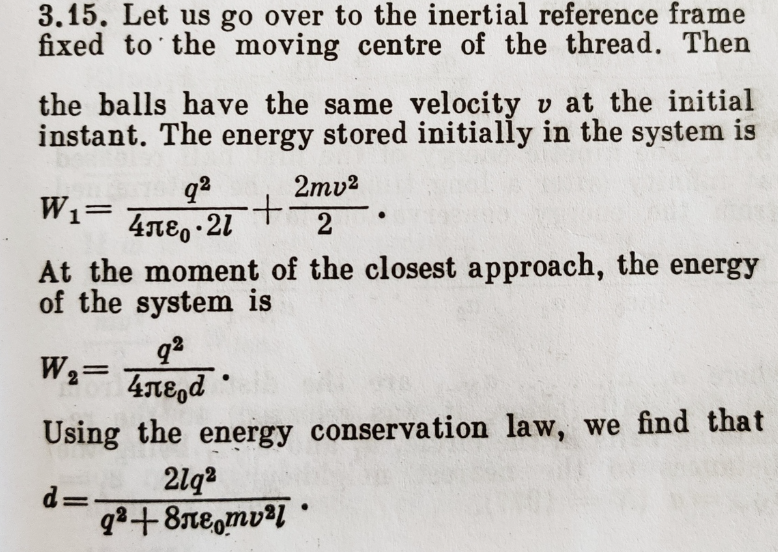- 1 year, 1 month ago

@Karan Chatrath I think conservation of energy is not working because while applying it, we can't say about the velocity of y direction of particles, but it 100% guaranteed that x direction velocity will be zero. Therefore I think the question is not as much easy as the author of the book thinks.

- 1 year, 1 month ago

You are right that it is not as easy as it looks. The answer provided in the solution is correct but the method used makes no sense. Hence my initial reservation about calling the book solution incorrect. But these extra checks have brought out some interesting features of this problem.

As to why the energy conservation principle does not work, it is not because of a lack of knowledge of the Y velocity components. I have used the Y velocity components while computing the final kinetic energy. I think that it does not work because the energy used to drive the system into motion is not accounted for. How that is done, I do not know yet.

The fact that the initial kinetic energy is zero and the final kinetic energy is not, completely contradicts the solution of the book.

- 1 year, 1 month ago

I believe that the book solution is correct. The author just decided to look at things from different reference frame and, since the problem assumes constant velocity of the middle of the thread, it's an inertial reference frame and all the laws of physics are completely valid. What more, this approach is more natural taking into account the way the problem is posed. He also correctly reasons that the velocity is 0 at the moment of minimum distance because the charged particles are constrained to move along the circle.

Regarding energy conservation, the equation obtained by @Karan Chatrath with respect to stationary observer is not correct because it's obvious that the energy is periodically provided to and taken away from the system in order to maintain the constant velocity of the thread. Energy in more general sense, seen as one of the integrals of equations of motion, equals: $\frac{\partial L}{\partial \dot{\theta}}\dot{\theta} - L = \text{const.}$ If one evaluates this expression using Lagrangian derived by @Steven Chase, one gets an equation identical to the one in the book.

- 1 year, 1 month ago

@Uros Stojkovic Sir but when author is applying energy conservation the velocity of particles in $x$ direction is zero and it should be. But the velocity in $y$ direction is not necessary to be zero, and the author has assumed it to be zero. So is it correct??

- 1 year, 1 month ago

It's correct. Velocity components (in the reference frame fixed at the middle of the thread) are dependent on each other because the motion of the charged particles is constrained to follow the circle of radius $l$.

- 1 year, 1 month ago

Another question is whether we are to assume that the velocity of the anchor point is constant. That is what I have assumed in my solution, but it is not clear whether that assumption is allowed. One thing we could do is just create a new problem with the following features:

1) The middle anchor point has constant upward velocity $v$
2) The balls are initially at rest
3) Each ball is connected to the anchor point by a rigid rod
4) The rods are hinged, and can rotate freely about the anchor point

If you set up the problem this way, I believe the dynamics are described by that equation with the secant/tangent. And we need not limit ourselves to small angles.

- 1 year, 1 month ago

Yes, I made the same assumptions when I worked out the equations of motion in Cartesian coordinates.

The rigid rod assumption is an interesting one and it aligns with the notion that the string remains taut throughout the motion.

Keeping in mind your related comment, I also agree that there is some form of external forcing. The question is then how can the work done by this external force be quantified. This is what eludes me.

- 1 year, 1 month ago

1) Give the anchor point mass
2) Remove the external forcing
3) Initialize the anchor point with some speed while the balls are at rest

I think that would be a nice problem with no ambiguity

- 1 year, 1 month ago

Sounds like a fun problem proposition. Would the anchor point only have an initial velocity $v$ along the Y direction, or would it have a constant velocity $v$ along the Y direction throughout time?

- 1 year, 1 month ago

It would only have initial velocity $v$. As the system evolved, that would change. Energy conservation would apply

- 1 year, 1 month ago

Sounds great. I would have offered to post it myself but I can only do so after several hours as I will be away from my laptop for the rest of my evening right until tomorrow. If you do post it in the meantime, I will get to it when I can.

- 1 year, 1 month ago

That's fine, I'll wait for you to post it. Should be fun

- 1 year, 1 month ago

@Karan Chatrath But is there any rule of staying away from laptop????

- 1 year, 1 month ago